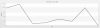## Recommended Posts

Hi folks.

I am experiencing problems creating a chart with more than one graphs from a php script. There are supposed to be three graphs in the chart but only one appears. Here's my XML data:

```<chart caption='15.01.2014 - 03.11.2014' subCaption='' showValues='0' useRoundEdges='1' showBorder='1' xAxisName='WoY' yAxisName='Revenue' decimals='2' formatNumberScale='0' numberSuffix=' €'>

<categories>
<category label='33' />
<category label='35' />
<category label='36' />
<category label='39' />
<category label='40' />
<category label='41' />
<category label='42' />
<category label='45' />
</categories>

<dataset seriesname='Total'>
<set value='1250.67' />
<set value='1522' />
<set value='150' />
<set value='195' />
<set value='470' />
<set value='300' />
<set value='1270' />
<set value='0' />
</dataset>

<dataset seriesname='Old' color='008ee4'>
<set value='500' />
<set value='1010' />
<set value='150' />
<set value='195' />
<set value='470' />
<set value='300' />
<set value='1270' />
<set value='0' />
</dataset>

<dataset seriesname='New' color='008ee4'>
<set value='750.67' />
<set value='512' />
<set value='0' />
<set value='0' />
<set value='0' />
<set value='0' />
<set value='0' />
<set value='0' />
</dataset>

</chart>
```

This XML data is stored in \$strXML and FusionCharts is called like this:

```<script type="text/javascript" src="fusioncharts/js/themes/fusioncharts.theme.zune.js"></script>
<script type="text/javascript">
var revenueChart = new FusionCharts({
"type": "line",
"renderAt": "chartContainer",
"width": "100%",
"height": "400",
"palette": "1",
"dataFormat": "xml",
"dataSource": "'.\$strXML.'"
});
revenueChart.render("chartContainer");
});
</script>';
```

The result can be seen in the attached PNG. What am I doing wrong?

Thank you all very much in advance!##### Share on other sites

Thank you so much, Moonmi! I totally missed that!